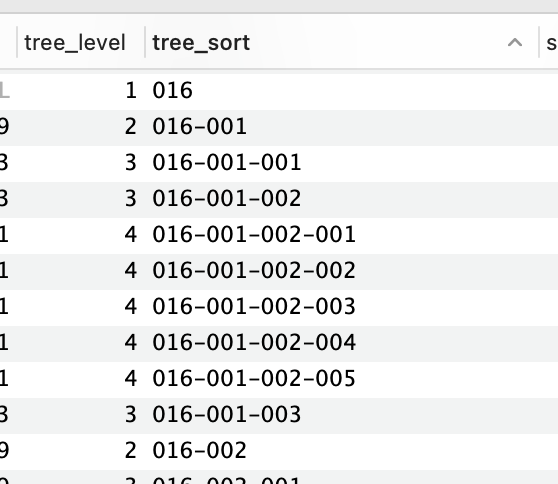# How I implimented a "Nested Treeview" with a table

I wanted to represent a network map in a table.. which consists of how our various datacenters/sites/customers are linked. Not sure if this is the best way to do it, but it works. (open to suggestions!)

## Each site is assigned a "tree_sort" ID so they can be sorted into position...So in RETOOL SQL query:

``````       select * from netsites
...
order by tree_sort ASC;
``````

## Add a Transformer to the Query to create the "nesting indents":

``````// Add NESTING Lines/Indentations to to names
return formatDataAsArray(data).map(row=>{
var n='';
let indent='&nbsp;&nbsp;&nbsp;';

if(row.tree_level>1){
n+='<span style="color:#ddd;">';

if(row.tree_level>2){   // if more than 1 indent, add vertical bars
let indent2=indent+'|'+indent;
n+=indent2.repeat(row.tree_level-2);
}

// now add the vert bar with horiz bar ie.  |--
n += indent + '|&#8212; </span>'
}
n += '<b>' +row.name + '</b> &nbsp; <span style="color:#ccc;">#'+row.netsite_id+'</span>';
row.tree_name2=n;
return row;
})
``````

## Here's the code in PHP Backend which creates the tree_sort ID from the Linked-List relationship

``````    public function calcTreeSort(){

// first reset all sites to the bottom of list (ie 999)
\$this->dbc->update("update donors set tree_sort='999', tree_level=''");

// get all root sites, but in case a name is missing (null), sort it to the bottom
\$q="select ROOT.id, ROOT.netsite_id, NS.name from netsite_root_ids ROOT
left join netsites NS USING (netsite_id)
order by ifnull(NS.name,'zzz') ASC";            // put nulls on bottom
\$res=\$this->dbc->select(\$q);

// setup sortcodes on each and recursively down the line
\$level=1;
\$currentSortCode=1;     //the current sort code resets at each tree level
foreach(\$res as \$r){
\$this->setSortCode_recurs(\$r->id, \$r->netsite_id, "", \$currentSortCode++, \$level);
}
}

// The sortPrefix is the current sites parent... (ie. "AAA-BBB-" is the parent of "AAA-BBB-CCC")
// currentSort is the current ID for this node
private function setSortCode_recurs(\$id, \$netsite_id, \$sortPrefix, \$currentSort, \$level, \$recurseLevel=1){
if(\$recurseLevel++ > 999) return;    //loop safety

// set current node

// update myself with propert sort code
\$q="update donors SET tree_sort=?, tree_level=? where id=?";
\$this->dbc->update(\$q, [\$mySortCode, \$level, \$id]);
echo str_repeat("   ",\$level-1) . "Level \$level: \$mySortCode    - set to \$id\n";

// get all child nodes, and update those
\$q="select D.id, D.netsite_id, NS.name from donors D
left join netsites NS USING (netsite_id)
WHERE D.`donor_netsite_id` = ?
order by NS.name ASC";
\$res=\$this->dbc->select(\$q, [\$netsite_id]);
if(\$res){
\$nextLevelSortCode=1;
\$level++;
\$nextPrefix = \$mySortCode . "-";
foreach(\$res as \$r){
\$this->setSortCode_recurs(\$r->id, \$r->netsite_id, \$nextPrefix, \$nextLevelSortCode++, \$level, \$recurseLevel);
}
}
}

``````

There's probably a better way to do, would love to hear other ideas...

5 Likes

Very creative!

Next level would be to add expand/collapse. Maybe have custom columns button with + and - icons. Clicking retransforms the underlying data.

``````001-001